×#### Thank you for registering.

One of our academic counsellors will contact you within 1 working day.

Click to Chat

1800-1023-196

+91-120-4616500

CART 0

• 0

MY CART (5)

Use Coupon: CART20 and get 20% off on all online Study Material

ITEM
DETAILS
MRP
DISCOUNT
FINAL PRICE
Total Price: Rs.

There are no items in this cart.
Continue ShoppingProperties of Gases and Gas Laws

Table of Content

General Properties of Gases

Parameters of Gases

Mass

Volume

Pressure

Temperature

Gas Laws

Boyle’s Law

Charles’s Law

Gay-Lussac’s Law or Amonation’s Law

Related ResourcesGeneral Properties of GasesGases do not have definite shape and volume

Gases occupy the whole space available to them.

Gases have unlimited dispensability and high compressibility.

They have very low densities because of negligible intermolecular forces.

Gases exert pressure on the walls of the container with perfectly elastic collisions.

They diffuse rapidly through each other from homogeneous mixture against the electric and gravitational field.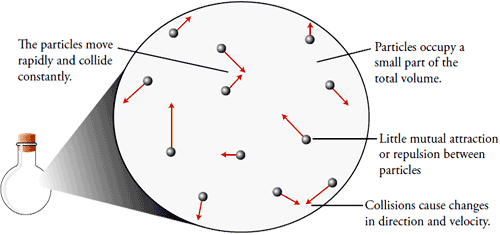Parameters of Gases

The characteristics of gases are described in terms of following four parameters

Mass

Volume

Pressure

Temperature

1. Mass (M)

The mass of the gas is related to the number of moles as

n = w/M

Where  n = number of moles

w = mass of gas in grams

M = molecular mass of the gas

2. Volume (V)

Since gases occupy the entire space available to them, therefore the gas volume means the volume of the container in which the gas is enclosed.

Units of Volume: Volume is generally expressed in litre (L), cm3 & dm3

1m3 = 103 litre = 103 dm3 = 106 cm3.

3. Pressure

Pressure of the gas is due to its collisions with walls of its container i.e. the force exerted by the gas per unit area on the walls of the container is equal to its pressure.

Pressure is exerted by a gas due to kinetic energy of its molecules.

As temperature increases, the kinetic energy of molecules increases, which results in increase in pressure of the gas. So, pressure of any gas is directly proportional to its temperature.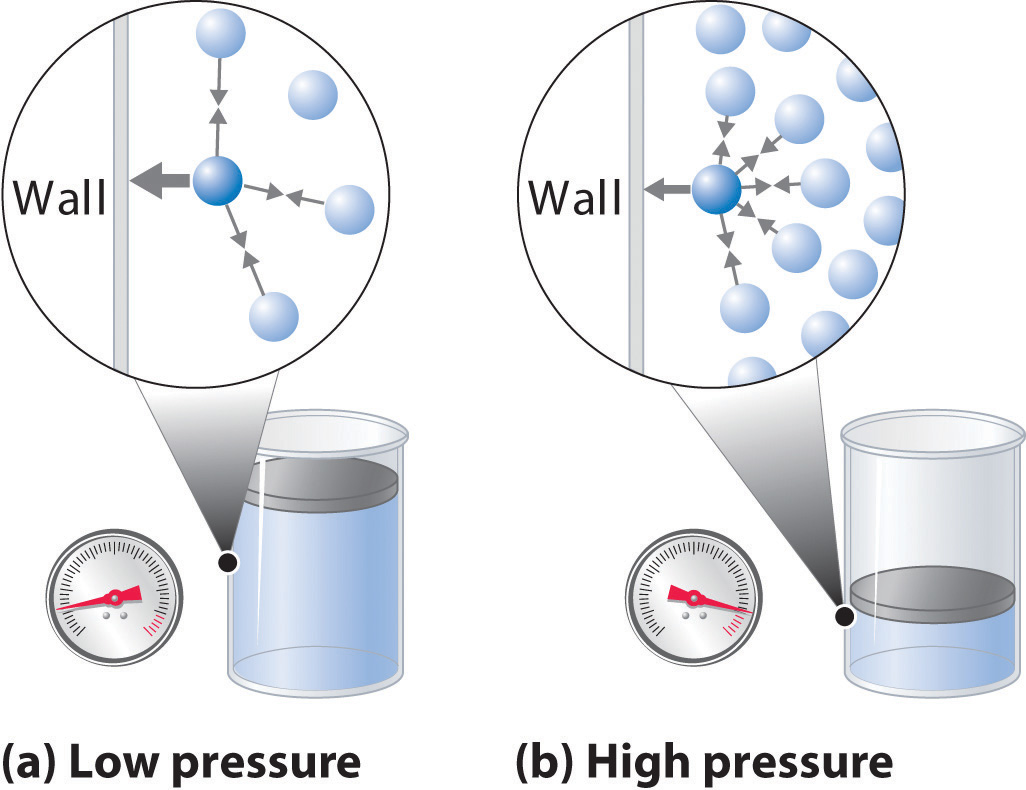Units of Pressure:

The pressure of a gas is expressed in atm, Pa, Nm–2, bar and lb/In2 (psi).

760 mm = 1 atm = 10132.5 KP­a = 101325 Pa = 101325 Nm–2

760 mm of Hg = 1.01325 bar = 1013.25 milli bar = 14.7 lb/2n2 (psi)

Watch this Video for more reference

4. Temperature (T):

Temperature is defined as the degree of hotness. The SI unit of temperature is Kelvin.  oC and oF are the two other units used for measuring temperature. On the Celsius scale water freezes at 0°C and boils at 100°C where as in the Kelvin scale water freezes at 273 K and boils at 373 K.

K = oC + 273.5

F = (9/5) oC + 32

Gas Laws

Boyle’s Law

In 1662, Robert Boyle discovered that there existed a relation between the pressure and the volume of a fixed amount of gas at a fixed temperature. In his experiment, he discovered that the product of Pressure & Volume of a fixed amount of gas at a fixed temperature was approximately a constant. So, Boyle’s low states that

”At constant temperature, the pressure of a fixed amount (i.e., number of moles n) of gas varies inversely with its volume”.Watch this Video for more reference

Mathematically

It means that at constant temperature, product of pressure and volume of a fixed amount of gas is constant.

If a fixed amount of gas at constant temperature T occupying volume V1 at pressure P1 undergoes expansion, so that volume becomes V2 and pressure becomes P2,
then according to Boyle’s law :

Now,

Using Boyle’s Law we get,

This indicates that at a constant temperature, pressure is directly proportional to the density of a fixed mass of the gas.

It is important to understand here the various units of pressure and their relationships.

The SI unit of pressure is Nm-2, which is called ‘Pascal’ (Pa) The pressure at sea level due to the weight of the earth’s atmosphere is approximately 105 Pa. Since pascal is a small unit, we express pressures in bar units, where 1 bar = 105 Pa. Atmospheric pressure is about one bar. Atmospheric pressure can also be expressed in atmosphere units abbreviated as atm.

1 atm = 1.01325 bar

Graphical Representation of Boyle’s Law :

A plot of P versus 1/V at constant temperature for a fixed mass of gas would be a straight line passing through the origin.

A plot of P versus V at constant temperature for a fixed mass of a gas would be a rectangular hyperbola.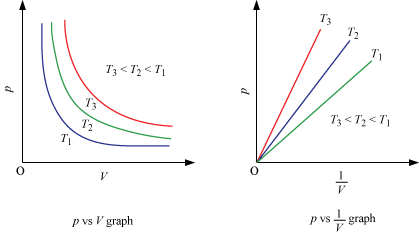A plot of P (or V ) versus PV at constant temperature for a fixed mass of a gas is a straight line parallel to the PV axis.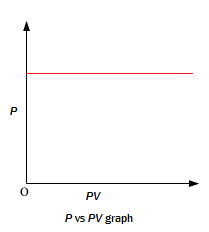Example 1:

Question:

A gas is present at a pressure of 2 atm. What should be the increase in pressure so that the volume of the gas can be decreased to ¼ th of the initial value, If the temperature is maintained constant?

Solution:

PV = Constant for a given mass of gas at constant pressure

P1V1 = P2V2

P1 = 2 atm

V1 = V

V2 = V/4

2 × V = P2 × V/4

P2 = 8atm

Pressure should be increased from  2 to 8 atm.

Total increase = 8-2 = 6 atm

Charles’s Law

In 1787, Jacques Charles discovered that if the pressure is kept constant, the volume of a gas sample increases linearly with the temperature for a fixed amount of gas. This law led to the idea of temperature. The unit of temperature used is Kelvin. Charles’s law states that

”At constant pressure, the volume of a given mass of a gas is directly proportional to its absolute temperature”Watch this Video for more reference

Mathematically

or

Hence, if  at constant pressure the volume of a gas V1  at temperature T1 change to V1  at  T2 we have

or

This equation is known as Charle’s Law equation or formula.

For each degree change in temperature, the volume of sample of a gas changes by the fraction of 1/273.5 of its volume at 0 oC.

So,

This equation is known as Charles-Gay-Lussac equation.

Where,

Vt = volume of gas at temperature t OC

V0 = volume of gas at 0 OC

T = temperature in OC

The temperature of -273 OC, at which the volume of a gas would theoretically be reduced to zero, is called absolute zero. At absolute zero temperature, the volume, pressure, kinetic energy and heat content of a gas is zero.

Graphical Representation of Charles’s Law :

1. For a definite mass of the gas a plot of V vs T (oK) at constant pressure is a straight line passing through the origin.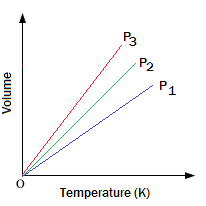2. A plot of V vs t (oC) at constant pressure is a straight line cutting the temperature axis at -273 oC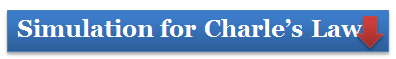Example 2:

Question:

Volume of given amount of a gas at 57 oC and constant pressure is 425.8 cm3. If the temperature is decreased to 37 oC at constant pressure, then what would be the volume of gas?

Solution:

According to Charle’s Law

Here

V1 = 425.8 cm3

V2 = ?

T1 =273+ 57 = 330 K

T2 =273+ 37 = 331 K

So,

Gay-Lussac’s Law or Amonation’s Law

This law states that “at constant volume, the pressure of a given mass of a gas is directly proportional to its absolute temperature”.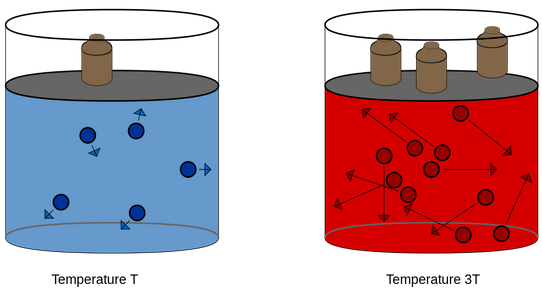Mathematically

Where,

P = Pressure of Gas

T= Absolute Temperature

If the pressure and temperature of a gas changes from P1 & T1 to P2 & T2 , volume remaining constant , we have

where,

Pt = Pressure of gas at t oC

Po = Pressure of gas at 0 oC

t = Temperature in oC.

Graphical Representation of Gay-Lussac’s Law“Samples of different gases which contain the same number of molecules (any complexity, size, shape) occupy the same volume at the same temperature and pressure”.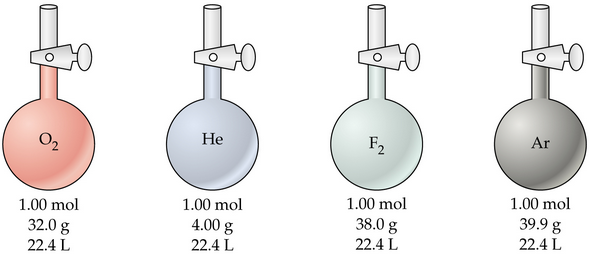It follows from Avogadro’s hypothesis that  (when T and P are constant).

Mathematically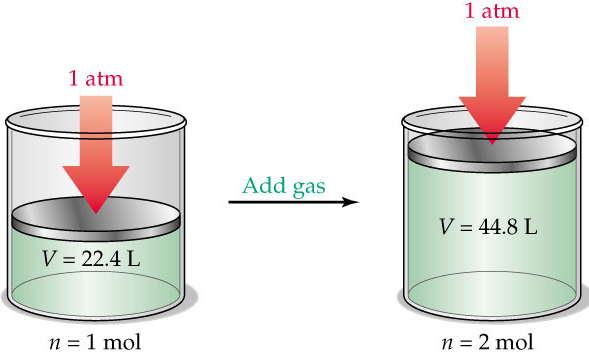Since volume of a gas is directly proportional to the number of moles; one mole of each gas at standard temperature and pressure (STP) will have same volume.
Standard temperature and pressure means 273.15 K (0°C) temperature and 1 bar (i.e., exactly 105 pascal) pressure. At STP molar volume of an ideal gas or a combination of ideal gases is 22.71098 L mol–1

We know that number of moles of any gas (n) =  m/M

Where m = mass of the gas under investigation and M = molar mass

Where, d = density of gas

So, we can conclude from equation  that the density of a gas is directly proportional to its molar mass.

A gas that follows Boyle’s law, Charles’ law and Avogadro law strictly is called an ideal gas. Such a gas is hypothetical. It is assumed that intermolecular forces are not present between the molecules of an ideal gas. Real gases follow these laws only under certain specific conditions when forces of interaction are practically negligible.Question 1: According to Charles’s Law

a. pV = nRT

b. V =kT

c. P = k/T

d. p1V1=p2V2

Question 2: Which of the following statements regarding gases is incorrect?

a. Gases do not have definite shape and volume

b. Gases occupy the whole space available to them.

c. Gases have unlimited dispensability and high compressibility.

d. They have very low densities because of  strong intermolecular forces.

Question 3: Pressure of the gas is due to

a. Collisions  of molecules with walls of container

b. Collisions  of molecules with another molecule

c. Random motion of molecules

d. Weak indermolecular forces

Question 4: What does the followIng plot represents?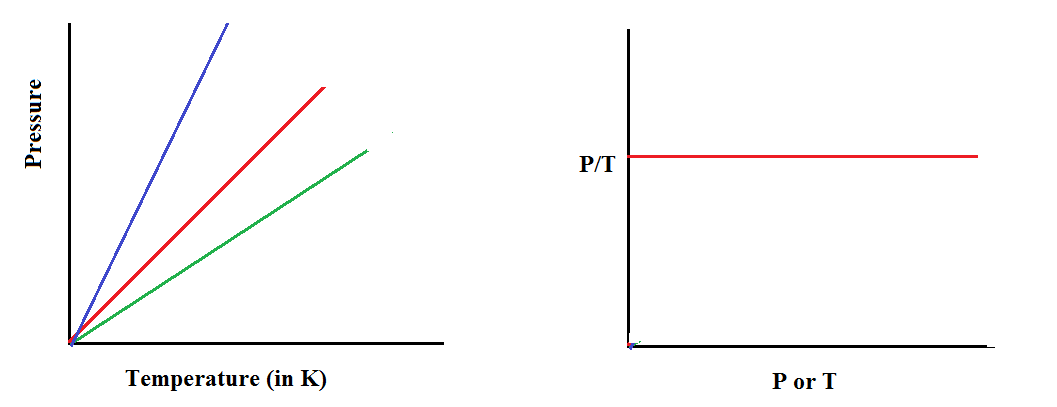a. Charles’s Law

b. Boyle’s Law

d. Gay-Lussac’s LawQ.1

Q.2

Q.3

Q.4

b

d

a

d

Related Resources

Look here for Reference books of  Chemistry

You can also refer States of matter

To read more, Buy study materials of States of Matter comprising study notes, revision notes, video lectures, previous year solved questions etc. Also browse for more study materials on Chemistry here.### Course Features

• 731 Video Lectures
• Revision Notes
• Previous Year Papers
• Mind Map
• Study Planner
• NCERT Solutions
• Discussion Forum
• Test paper with Video Solution